1561177675

# Learn NumPy Arrays With Examples

So, let’s get started!

## What is a Python NumPy?

NumPy is a Python package which stands for ‘Numerical Python’. It is the core library for scientific computing, which contains a powerful n-dimensional array object, provide tools for integrating C, C++ etc. It is also useful in linear algebra, random number capability etc. NumPy array can also be used as an efficient multi-dimensional container for generic data. Now, let me tell you what exactly is a python numpy array.

**NumPy Array: **Numpy array is a powerful N-dimensional array object which is in the form of rows and columns. We can initialize numpy arrays from nested Python lists and access it elements. In order to perform these numpy operations, the next question which will come in your mind is:

## How do I install NumPy?

To install Python NumPy, go to your command prompt and type “pip install numpy”. Once the installation is completed, go to your IDE (For example: PyCharm) and simply import it by typing: “import numpy as np”

Moving ahead in** python numpy tutorial**, let us understand what exactly is a multi-dimensional numPy array.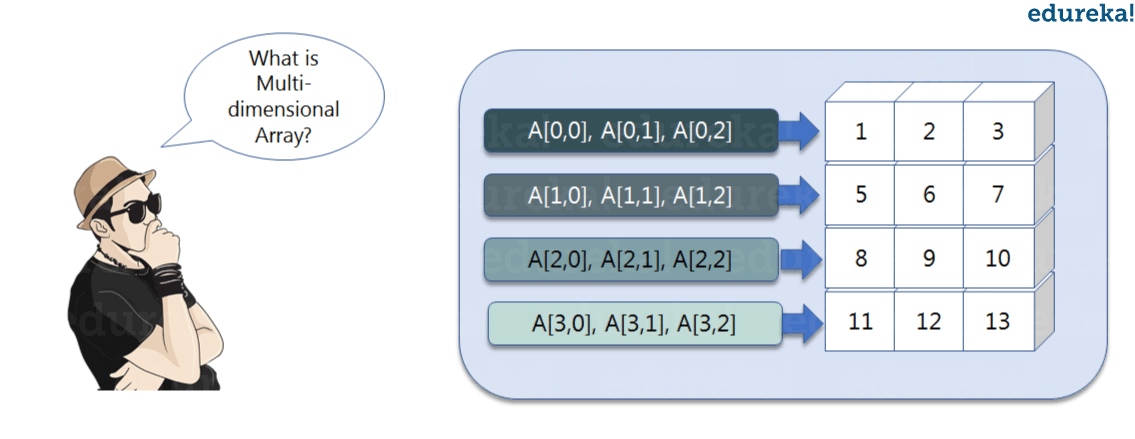Here, I have different elements that are stored in their respective memory locations. It is said to be two dimensional because it has rows as well as columns. In the above image, we have 3 columns and 4 rows available.

Let us see how it is implemented in PyCharm:

### Single-dimensional Numpy Array:

``````import numpy as np
a=np.array([1,2,3])
print(a)

``````
``````Output – [1 2 3]

``````

### Multi-dimensional Array:

``````a=np.array([(1,2,3),(4,5,6)])
print(a)

``````
``````O/P – [[ 1 2 3]
[4 5 6]]

``````

Many of you must be wondering that why do we use python numpy if we already have python list? So, let us understand with some examples in this python numpy tutorial.

## Python NumPy Array v/s List

We use python numpy array instead of a list because of the below three reasons:
Less MemoryFastConvenient
The very first reason to choose python numpy array is that it occupies less memory as compared to list. Then, it is pretty fast in terms of execution and at the same time it is very convenient to work with numpy. So these are the major advantages that python numpy array has over list. Don’t worry, I am going to prove the above points one by one practically in PyCharm. Consider the below example:

``````import numpy as np

import time
import sys
S= range(1000)
print(sys.getsizeof(5)*len(S))

D= np.arange(1000)
print(D.size*D.itemsize)

``````
``````O/P –  14000
4000

``````

The above output shows that the memory allocated by list (denoted by S) is 14000 whereas the memory allocated by the numpy array is just 4000. From this, you can conclude that there is a major difference between the two and this makes python numpy array as the preferred choice over list.

Next, let’s talk how python numpy array is faster and more convenient when compared to list.

``````import time
import sys

SIZE = 1000000

L1= range(SIZE)
L2= range(SIZE)
A1= np.arange(SIZE)
A2=np.arange(SIZE)

start= time.time()
result=[(x,y) for x,y in zip(L1,L2)]
print((time.time()-start)*1000)

start=time.time()
result= A1+A2
print((time.time()-start)*1000)

``````
``````O/P – 380.9998035430908
49.99995231628418

``````

In the above code, we have defined two lists and two numpy arrays. Then, we have compared the time taken in order to find the sum of lists and sum of numpy arrays both. If you see the output of the above program, there is a significant change in the two values. List took 380ms whereas the numpy array took almost 49ms. Hence, numpy array is faster than list. Now, if you noticed we had run a ‘for’ loop for a list which returns the concatenation of both the lists whereas for numpy arrays, we have just added the two array by simply printing A1+A2. That’s why working with numpy is much easier and convenient when compared to the lists.

Therefore, the above examples proves the point as to why you should go for python numpy array rather than a list!

Moving forward in python numpy tutorial, let’s focus on some of its operations.

## Python NumPy Operations

ndim:
You can find the dimension of the array, whether it is a two-dimensional array or a single dimensional array. So, let us see this practically how we can find the dimensions. In the below code, with the help of ‘ndim’ function, I can find whether the array is of single dimension or multi dimension.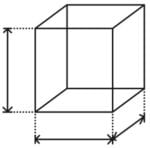``````import numpy as np
a = np.array([(1,2,3),(4,5,6)])
print(a.ndim)

``````
``````Output – 2

``````

Since the output is 2, it is a two-dimensional array (multi dimension).

• itemsize:

You can calculate the byte size of each element. In the below code, I have defined a single dimensional array and with the help of ‘itemsize’ function, we can find the size of each element.``````import numpy as np
a = np.array([(1,2,3)])
print(a.itemsize)

``````
``````Output – 4

``````

So every element occupies 4 byte in the above numpy array.

• dtype:

You can find the data type of the elements that are stored in an array. So, if you want to know the data type of a particular element, you can use ‘dtype’ function which will print the datatype along with the size. In the below code, I have defined an array where I have used the same function.``````import numpy as np
a = np.array([(1,2,3)])
print(a.dtype)

``````
``````Output – int32

``````

As you can see, the data type of the array is integer 32 bits. Similarly, you can find the size and shape of the array using ‘size’ and ‘shape’ function respectively.

``````import numpy as np
a = np.array([(1,2,3,4,5,6)])
print(a.size)
print(a.shape)

``````
``````Output – 6 (1,6)

``````

Next, let us move forward and see what are the other operations that you can perform with python numpy module. We can also perform reshape as well as slicing operation using** python numpy operation**. But, what exactly is reshape and slicing? So let me explain this one by one in this python numpy tutorial.
reshape:
Reshape is when you change the number of rows and columns which gives a new view to an object. Now, let us take an example to reshape the below array: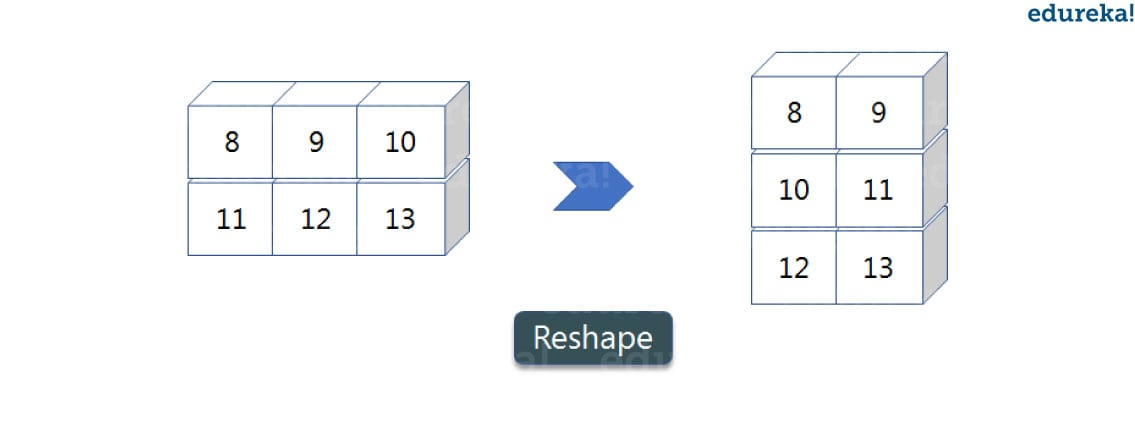As you can see in the above image, we have 3 columns and 2 rows which has converted into 2 columns and 3 rows. Let me show you practically how it’s done.

``````import numpy as np
a = np.array([(8,9,10),(11,12,13)])
print(a)
a=a.reshape(3,2)
print(a)

``````
``````Output – [[ 8 9 10] [11 12 13]] [[ 8 9] [10 11] [12 13]]

``````
• slicing:

As you can see the ‘reshape’ function has showed its magic. Now, let’s take another operation i.e Slicing. Slicing is basically extracting particular set of elements from an array. This slicing operation is pretty much similar to the one which is there in the list as well. Consider the following example: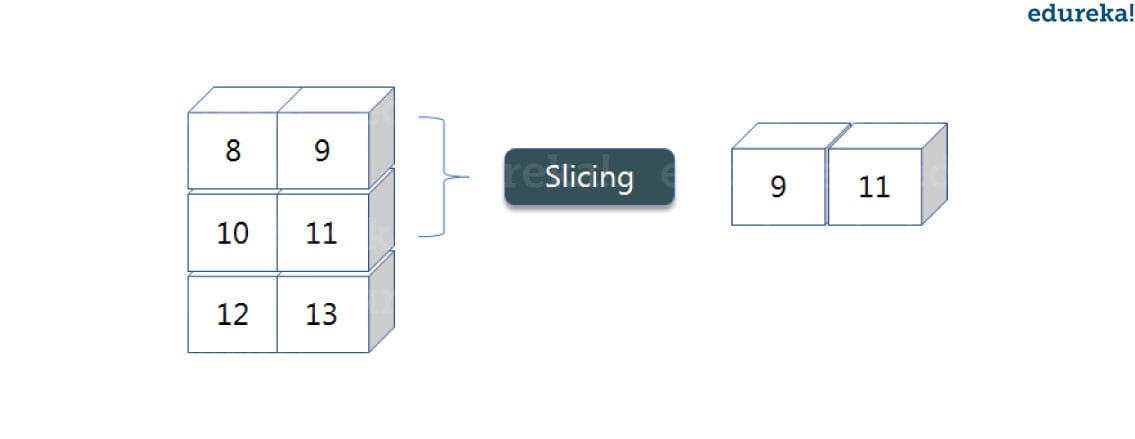Before getting into the above example, let’s see a simple one. We have an array and we need a particular element (say 3) out of a given array. Let’s consider the below example:

``````import numpy as np
a=np.array([(1,2,3,4),(3,4,5,6)])
print(a[0,2])

``````

Output – 3

Here, the array(1,2,3,4) is your index 0 and (3,4,5,6) is index 1 of the python numpy array. Therefore, we have printed the second element from the zeroth index.

Taking one step forward, let’s say we need the 2nd element from the zeroth and first index of the array. Let’s see how you can perform this operation:

``````import numpy as np
a=np.array([(1,2,3,4),(3,4,5,6)])
print(a[0:,2])

``````

Output – [3 5]

Here colon represents all the rows, including zero. Now to get the 2nd element, we’ll call index 2 from both of the rows which gives us the value 3 and 5 respectively.

Next, just to remove the confusion, let’s say we have one more row and we don’t want to get its 2nd element printed just as the image above. What we can do in such case?

Consider the below code:

``````import numpy as np
a=np.array([(8,9),(10,11),(12,13)])
print(a[0:2,1])

``````

Output – [9 11]

As you can see in the above code, only 9 and 11 gets printed. Now when I have written 0:2, this does not include the second index of the third row of an array. Therefore, only 9 and 11 gets printed else you will get all the elements i.e [9 11 13].
linspace
This is another operation in python numpy which returns evenly spaced numbers over a specified interval. Consider the below example:

``````import numpy as np
a=np.linspace(1,3,10)
print(a)

``````

Output – [ 1. 1.22222222 1.44444444 1.66666667 1.88888889 2.11111111 2.33333333 2.55555556 2.77777778 3. ]

As you can see in the result, it has printed 10 values between 1 to 3.
max/ min
Next, we have some more operations in numpy such as to find the minimum, maximum as well the sum of the numpy array. Let’s go ahead in python numpy tutorial and execute it practically.

``````import numpy as np

a= np.array([1,2,3])
print(a.min())
print(a.max())
print(a.sum())

``````

Output – 1 3 6

You must be finding these pretty basic, but with the help of this knowledge you can perform a lot bigger tasks as well. Now, lets understand the concept of axis in python numpy.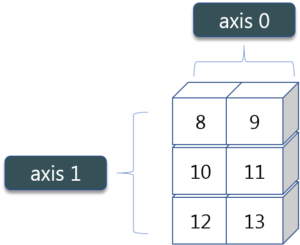As you can see in the figure, we have a numpy array 2*3. Here the rows are called as axis 1 and the columns are called as axis 0. Now you must be wondering what is the use of these axis?

Suppose you want to calculate the sum of all the columns, then you can make use of axis. Let me show you practically, how you can implement axis in your PyCharm:

``````a= np.array([(1,2,3),(3,4,5)])
print(a.sum(axis=0))

``````

Output – [4 6 8]

Therefore, the sum of all the columns are added where 1+3=4, 2+4=6 and 3+5=8. Similarly, if you replace the axis by 1, then it will print [6 12] where all the rows get added.
Square Root & Standard Deviation
There are various mathematical functions that can be performed using python numpy. You can find the square root, standard deviation of the array. So, let’s implement these operations:

``````import numpy as np
a=np.array([(1,2,3),(3,4,5,)])
print(np.sqrt(a))
print(np.std(a))

``````

Output – [[ 1. 1.41421356 1.73205081]

[ 1.73205081 2. 2.23606798]]

1.29099444874

As you can see the output above, the square root of all the elements are printed. Also, the standard deviation is printed for the above array i.e how much each element varies from the mean value of the python numpy array.
You can perform more operations on numpy array i.e addition, subtraction,multiplication and division of the two matrices. Let me go ahead in python numpy tutorial, and show it to you practically:

``````import numpy as np
x= np.array([(1,2,3),(3,4,5)])
y= np.array([(1,2,3),(3,4,5)])
print(x+y)

``````

Output – [[ 2 4 6] [ 6 8 10]]

This is extremely simple! Right? Similarly, we can perform other operations such as subtraction, multiplication and division. Consider the below example:

``````import numpy as np
x= np.array([(1,2,3),(3,4,5)])
y= np.array([(1,2,3),(3,4,5)])
print(x-y)
print(x*y)
print(x/y)

``````

Output – [[0 0 0] [0 0 0]]

[[ 1 4 9] [ 9 16 25]]

[[ 1. 1. 1.] [ 1. 1. 1.]]
Vertical & Horizontal Stacking
Next, if you want to concatenate two arrays and not just add them, you can perform it using two ways – vertical stacking and horizontal stacking. Let me show it one by one in this python numpy tutorial.

``````import numpy as np
x= np.array([(1,2,3),(3,4,5)])
y= np.array([(1,2,3),(3,4,5)])
print(np.vstack((x,y)))
print(np.hstack((x,y)))

``````

Output – [[1 2 3] [3 4 5] [1 2 3] [3 4 5]]

[[1 2 3 1 2 3] [3 4 5 3 4 5]]
ravel
There is one more operation where you can convert one numpy array into a single column i.e ravel. Let me show how it is implemented practically:

``````import numpy as np
x= np.array([(1,2,3),(3,4,5)])
print(x.ravel())

``````

Output – [ 1 2 3 3 4 5]

Let’s move forward in python numpy tutorial, and look at some of its special functions.

## Python Numpy Special Functions

There are various special functions available in numpy such as sine, cosine, tan, log etc. First, let’s begin with sine function where we will learn to plot its graph. For that, we need to import a module called matplotlib. To understand the basics and practical implementations of this module, you can refer Matplotlib Tutorial. Moving ahead with python numpy tutorial, let’s see how these graphs are plotted.

``````import numpy as np
import matplotlib.pyplot as plt
x= np.arange(0,3*np.pi,0.1)
y=np.sin(x)
plt.plot(x,y)
plt.show()

``````

Output –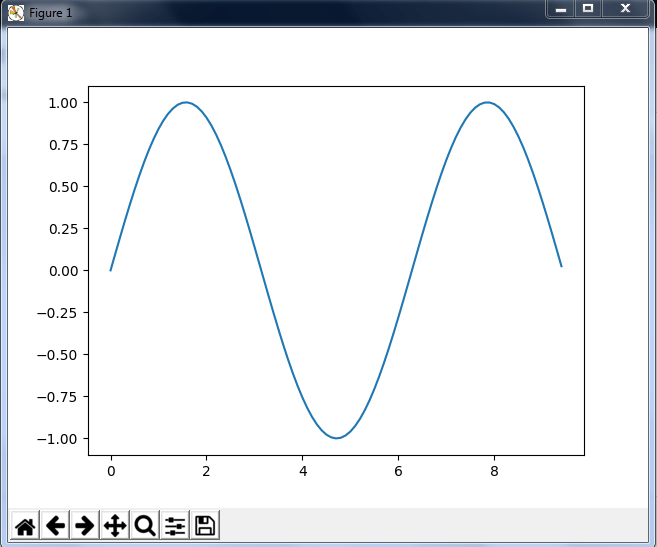Similarly, you can plot a graph for any trigonometric function such as cos, tan etc. Let me show you one more example where you can plot a graph of another function, let’s say tan*.*

``````import numpy as np
import matplotlib.pyplot as plt
x= np.arange(0,3*np.pi,0.1)
y=np.tan(x)
plt.plot(x,y)
plt.show()

``````

Output –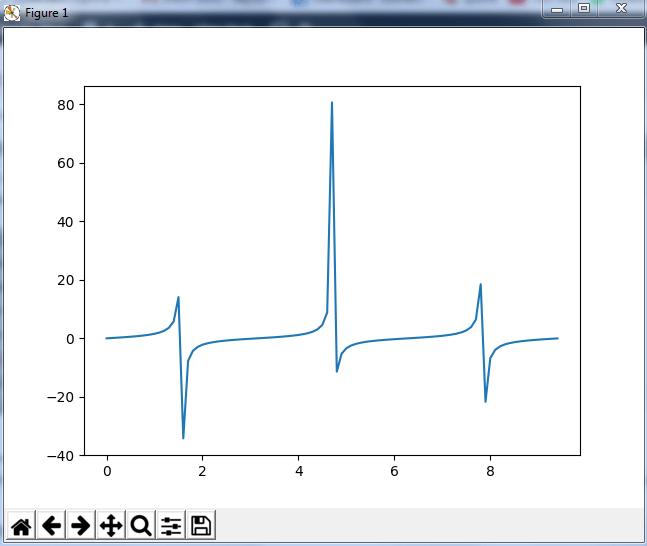Moving forward with python numpy tutorial, let’s see some other special functionality in numpy array such as exponential and logarithmic function. Now in exponential, the *e *value is somewhere equal to 2.7 and in log, it is actually log base 10. When we talk about natural log i.e log base e, it is referred as Ln. So let’s see how it is implemented practically:

``````a= np.array([1,2,3])
print(np.exp(a))

``````

Output – [ 2.71828183 7.3890561 20.08553692]

As you can see the above output, the exponential values are printed i.e e raise to the power 1 is e, which gives the result as 2.718… Similarly, e raise to the power of 2 gives the value somewhere near 7.38 and so on. Next, in order to calculate log, let’s see how you can implement it:

``````import numpy as np
import matplotlib.pyplot as plt
a= np.array([1,2,3])
print(np.log(a))

``````

Output – [ 0. 0.69314718 1.09861229]

Here, we have calculated natural log which gives the value as displayed above. Now, if we want log base 10 instead of Ln or natural log, you can follow the below code:

``````import numpy as np
import matplotlib.pyplot as plt
a= np.array([1,2,3])
print(np.log10(a))

``````

Output – [ 0. 0.30103 0.47712125]

By this, we come to the end of this python numpy tutorial. We have covered all the basics of python numpy, so you can start practicing now. The more you practice, the more you will learn.

#python #numpy

## Buddha Community1595467140

## NumPy Array Tutorial - Python NumPy Array Operations and Methods

The most important feature of NumPy is the homogeneous high-performance n-dimensional array object. Data manipulation in Python is nearly equivalent to the manipulation of NumPy arrays. NumPy array manipulation is basically related to accessing data and sub-arrays. It also includes array splitting, reshaping, and joining of arrays. Even the other external libraries in Python relate to NumPy arrays.

_Keeping you updated with latest technology trends, _Join DataFlair on Telegram

## Numpy Array Basics

Arrays in NumPy are synonymous with lists in Python with a homogenous nature. The homogeneity helps to perform smoother mathematical operations. These arrays are mutable. NumPy is useful to perform basic operations like finding the dimensions, the bite-size, and also the data types of elements of the array.

## NumPy Array Creation

### 1. Using the NumPy functions

NumPy has a variety of built-in functions to create an array.

#### a. Creating one-dimensional array in NumPy

For 1-D arrays the most common function is np.arange(…), passing any value create an array from 0 to that number.

1. import numpy as np
2. array=np.arange(20)
3. array

Output

array([ 0, 1, 2, 3, 4, 5, 6, 7, 8, 9, 10, 11,12, 13, 14, 15, 16, 17, 18, 19])

We can check the dimensions by using array.shape.

#numpy tutorials #array in numpy #numpy array #python numpy array1616074200

## The Basics of Numpy Arrays

Data manipulation in Python is nearly synonymous with Numpy array manipulation, even newer tools like Pandas are built around the Numpy array. This section will present several examples using Numpy and manipulation to access data and subarrays, and to split, reshape and join arrays.
Let’s start by defining three random arrays: a one-dimensional, two-dimensional, and three dimensional array. We’ll use Numpy’s random number generator, which we will seed with a set value in order to ensure that the same random arrays are generated each time this code is run.

#data-science #numpy #numpy-tutorial #numpy-array #learning1619667660

## Computation on Numpy Arrays:

Up until now, we have been discussing some of the basic nuts and bolts of Numpy ; in this section we will dive deep into the reasons that Numpy is so important in the Python Data Science world.

The key to make the computation on Numpy arrays fast is to use vectorized operations, generally implemented through Numpy’s Universal functions (ufuncs). The vectorized approach is designed to push loop into the compiled layer that underlies Numpy, leading to much faster execution. Vectorized operations in Numpy are implemented via ufuncs, whose main purpose is to quickly execute repeated operations on values in Numpy arrays.

#numpy #numpy-array #learning #data-science #learning-to-code1619660285

## Linear Algebra for Data Scientists with NumPy - Analytics India Magazine

A geek in Machine Learning with a Master’s degree in…###### Delhivery Promises To Fly Charters With Oxygen Concentrators In India

NumPy is an essential Python library to perform mathematical and scientific computations. NumPy offers Python’s array-like data structures with exclusive operations and methods. Many data science libraries and frameworks, including PandasScikit-Learn, Statsmodels, Matplotlib and SciPy, are built on top of NumPy with Numpy arrays in their building blocks. Some frameworks, including TensorFlow and PyTorch, introduce NumPy arrays or NumPy-alike arrays as their fundamental data structure in the name of tensors.How NumPy becomes the base of Data Science computing system (source)

Data Science relies heavily on Linear Algebra. NumPy is famous for its Linear Algebra operations. This article discusses methods available in the NumPy library to perform various Linear Algebra operations with examples. These examples assume that the readers have a basic understanding of NumPy arrays. Check out the following articles to have a better understanding of NumPy fundamentals:

#developers corner #linear algebra #matrices #numpy #numpy array #numpy dot product #numpy matrix multiplication #numpy tutorial #svd #vectors1595235240

## NumPy Applications - Uses of Numpy

In this Numpy tutorial, we will learn Numpy applications.

NumPy is a basic level external library in Python used for complex mathematical operations. NumPy overcomes slower executions with the use of multi-dimensional array objects. It has built-in functions for manipulating arrays. We can convert different algorithms to can into functions for applying on arrays.NumPy has applications that are not only limited to itself. It is a very diverse library and has a wide range of applications in other sectors. Numpy can be put to use along with Data Science, Data Analysis and Machine Learning. It is also a base for other python libraries. These libraries use the functionalities in NumPy to increase their capabilities.#### 1. An alternative for lists and arrays in Python

Arrays in Numpy are equivalent to lists in python. Like lists in python, the Numpy arrays are homogenous sets of elements. The most important feature of NumPy arrays is they are homogenous in nature. This differentiates them from python arrays. It maintains uniformity for mathematical operations that would not be possible with heterogeneous elements. Another benefit of using NumPy arrays is there are a large number of functions that are applicable to these arrays. These functions could not be performed when applied to python arrays due to their heterogeneous nature.

#### 2. NumPy maintains minimal memory

Arrays in NumPy are objects. Python deletes and creates these objects continually, as per the requirements. Hence, the memory allocation is less as compared to Python lists. NumPy has features to avoid memory wastage in the data buffer. It consists of functions like copies, view, and indexing that helps in saving a lot of memory. Indexing helps to return the view of the original array, that implements reuse of the data. It also specifies the data type of the elements which leads to code optimization.

#### 3. Using NumPy for multi-dimensional arrays

We can also create multi-dimensional arrays in NumPy.These arrays have multiple rows and columns. These arrays have more than one column that makes these multi-dimensional. Multi-dimensional array implements the creation of matrices. These matrices are easy to work with. With the use of matrices the code also becomes memory efficient. We have a matrix module to perform various operations on these matrices.

#### 4. Mathematical operations with NumPy

Working with NumPy also includes easy to use functions for mathematical computations on the array data set. We have many modules for performing basic and special mathematical functions in NumPy. There are functions for Linear Algebra, bitwise operations, Fourier transform, arithmetic operations, string operations, etc.

#numpy tutorials #applications of numpy #numpy applications #uses of numpy #numpy